PRICE BOOK VALUE MULTIPLES

General Issues in estimating and using price-book value ratios

Measurement

The book value of equity is the difference between the book value of assets and the book value of liabilities.

The measurement of the book value of assets is largely determined by accounting convention.

Book Value versus Market Value

The market value of an asset reflects its earning power and expected cashflows.

Since the book value of an asset reflects its original cost, it might deviate significantly from market value if the earning power of the asset has increased or declined significantly since its acquisition.

Advantages of using price/book value ratios

It provides a relatively stable, intuitive measure of value which can be compared to the market price.

Given reasonably consistent accounting standards across firms, price-book value ratios can be compared across similar firms for signs of under or over valuation.

Even firms with negative earnings, which cannot be valued using PE ratios, can be evaluated using price-book value ratios.

Disadvantages of using price-book value ratios

Book values, like earnings, are affected by accounting decisions on depreciation and other variables. When accounting standards vary widely across firms, the price-book value ratios may not be comparable across firms.

Book value may not carry much meaning for service firms which do not have significant fixed assets.

The book value of equity can become negative if a firm has a sustained string of negative earnings reports, leading to a negative price-book value ratio.

Estimating price-book value ratios from fundamentals

PBV Ratio for a stable firm

The value of equity for a stable firm, using the Gordon growth model is: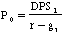Defining the return on equity (ROE) = EPS0 / Book Value of Equity, the value of equity can be written as: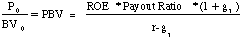If the return on equity is based upon expected earnings in the next time period, this can be simplified to,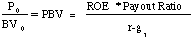A Formulation based upon differential returns

This formulation can be simplified even further by relating growth to the return on equity:

g = (1 - Payout ratio) * ROE

Substituting back into the P/BV equation,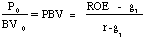The price-book value ratio of a stable firm is determined by the differential between the return on equity and the required rate of return on its projects.

Illustration 13: Estimating the PBV ratio for a stable firm with dividends- Amoco

Amoco had earnings per share of \$3.82 in 1994, and paid out 60% of its earnings as dividends that year. The growth rate in earnings and dividends, in the long term, is expected to be 6%. The return on equity at Amoco in 1994 was 15%. The beta for Amoco is 0.65 and the treasury bond rate is 7.5%.

Current Dividend Payout Ratio = 60%

Expected Growth Rate in Earnings and Dividends = 6%

Return on Equity =15%

Cost of Equity = 7.5% + 0.65*5.5% = 11.08 %

PBV Ratio based on fundamentals = 0.15 * 0.60 *1.06 / (.1108 -.06) = 1.88

PBV Ratio based upon return differential = (0.15 - 0.06) / (0.1108 - 0.06) = 1.77

Amoco was selling at a P/BV ratio of just about 2.00 on the day of this analysis. (March 1995)

P/BV and ROE for Oil Companies - 1995

 Company Price per Share BV per share Price/BV ROE Elf Aquitane 36 29.6 1.22 5.0% Amerada Hess 45 32.65 1.38 3.5% Getty 12 8.15 1.47 11.0% Murphy Oil 42 27.95 1.50 7.5% Ashland Oil 34 21.23 1.60 12.4% Repsol 27 15.35 1.76 15.5% Royal Dutch 107 58.95 1.82 12.0% Texaco 61 33.15 1.84 11.0% Occidental Pete 19 9.95 1.91 9.5% Mobil 85 44.5 1.91 13.0% Chevron Corp 44 22.2 1.98 13.0% Amoco 59 28.85 2.05 15.0% Exxon Corp 62 28.3 2.19 15.0% British Petroleum 80 33.6 2.38 15.0% Unocal 27 11.3 2.39 13.0% Atlantic Richfield 103 38.9 2.65

OIL COMPANIES: P/BV AND ROE

Correlation between PBV Ratios and ROE = 0.78

Regression: PBV = 0.91 + 8.26 (ROE) R2 = 0.61

Valuing a Privatization Candidate (Oil)

Assume that you have been asked to value a PEMEX for the Mexican Government; All you know is that it has earned a return on equity of 14% last year. The appropriate P/BV ratio can be estimated in one of two ways ñ

Beta based upon international oil companies = 0.70

Cost of Equity = 7.50% + 0.70 (5.50%) = 11.35%

P/BV Ratio (based upon fundamentals) = (0.14 - 0.06) / (.1135 - 0.06) = 1.50

P/BV Ratio (based upon regression) = 0.91 + 8.26 * 0.14 = 2.07

Illustration 14: Estimating the price-book value ratio for a 'privatization' candidate - Jenapharm (Germany)

Jenapharm was the most respected pharmaceutical manufacturer in East Germany.

Jenapharm, which was expected to have revenues of 230 million DM in 1991, also was expected to make earnings before interest and taxes of 30 million DM.

The firm had a book value of assets of 110 million DM, and a book value of equity of 58 million DM. The interest expenses in 1990 amounted to 15 million DM.

The firm was expected to maintain sales in its niche product, a contraceptive pill, and grow at 5% a year in the long term, primarily by expanding into the generic drug market.

The average beta of pharmaceutical firms traded on the Frankfurt Stock exchange wass 1.05, though many of these firms had much more diversified product portfolios and less volatile cashflows.

Allowing for the higher leverage and risk in Jenapharm, a beta of 1.25 was used for Jenapharm. The ten-year bond rate in Germany at the time of this valuation was 7%, and the risk premium for stocks over bonds is assumed to be 3.5%.

Valuing Jenapharm

Expected Net Income = (EBIT - Interest Expense)*(1-t) = (30 - 15) *(1-0.4) = 9 mil DM

Return on Equity = Expected Net Income / Book Value of Equity = 9 / 58 = 15.52%

Cost on Equity = 7% + 1.25 (3.5%) = 11.375%

Price/Book Value Ratio = (ROE - g) / (r - g) = (.1552 - .05) / (.11375 - .05) = 1.65

Estimated MV of equity = BV of Equity * Price/BV ratio = 58 * 1.65 = 95.70 mil DM

PBV Ratio for a high growth firm

When the growth rate is assumed to be constant after the initial high growth phase, the dividend discount model can be written as follows: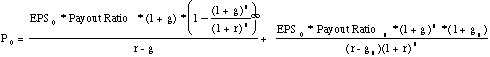Rewriting EPS0 in terms of the return on equity, EPS0 = BV0*ROE, and bringing BV0 to the left hand side of the equation, we getIllustration 15: Estimating the PBV ratio for a high growth firm in the two-stage model

Assume that you have been asked to estimate the PBV ratio for a firm which has the following characteristics:

Growth rate in first five years = 20% Payout ratio in first five years = 20%

Growth rate after five years = 8% Payout ratio after five years = 68%

Beta = 1.0 Riskfree rate = T.Bond Rate = 6%

Return on equity = 25%

Required rate of return = 6% + 1(5.5%)= 11.5%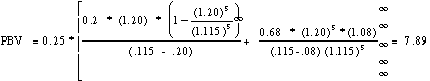The estimated PBV ratio for this firm is 7.89.

Illustration 16: Estimating the Price/Book Value Ratio for a high growth firm - Bertelsmann AG

Bertelsmann AG is a German publishing company which is involved in a wide range of media.

High Growth Period Stable Growth Period

Expected length = 10 years Growth Rate = 6%

Growth Rate = 20% Payout Ratio = 60%

Payout Ratio = 30% Return on Equity = 15%

Return on Equity = 28.57%

Beta in both periods is 0.90. The German long bond rate was approximately 7.50%.

Note: Expected Growth Rate = (1 - Payout Ratio) (ROE)

Cost of Equity = 7.50% + 0.90 * 5.5% = 12.45 %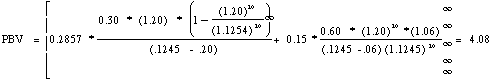PBV Ratios and Return on Equity

The ratio of price to book value is strongly influenced by the return on equity.

A lower return on equity affects the price-book value ratio directly through the formulation specified in the prior section and

indirectly, by lowering the expected growth or payout.

Expected growth rate = Retention Ratio * Return on Equity

Illustration 17: Return on Equity and Price-Book Value

Assume that a firm has the following characteristics:

Return on Equity = 25%

Growth rate in first five years= 20% Payout ratio in first five years =20%

Growth rate after five years = 8% Payout ratio after five years = 68 %

Beta = 1.0 Required rate of return = 11.5%

Note that the growth rate in first five years = Retention ratio * ROE = 0.8 * 25% = 20%

and the growth rate after year 5 = Retention ratio * ROE = 0.32 * 25% = 8%Effect of a Drop in the ROE

If the firm's return on equity drops to 12%, the price/book value will reflect the drop. The lower return on equity will also lower expected growth in the initial high growth period:

Expected growth rate (first five years) = Retention ratio * Return on Equity

= 0.80* 12% = 9.6%

After year 5, either the retention ratio has to increase or the expected growth rate has to be lower than 8%. If the retention ratio is adjusted,

New retention ratio after year 5 = Expected growth / ROE = 8%/12% = 66.67%

New payout ratio after year 5 = 1 - Retention ratio = 33.33%

The new price-book value ratio can then be calculated as follows:The drop in the ROE has a two-layered impact. First, it lowers the growth rate in earnings and/or the expected payout ratio, thus having an indirect effect on the P/BV ratio. Second, it reduces the P/BV ratio directly.Note that when the return on equity is equal to the required return, the price is equal to the book value.Looking for undervalued securities - PBV Ratios and ROE

• Given the relationship between price-book value ratios and returns on equity, it is not surprising to see firms which have high returns on equity selling for well above book value and firms which have low returns on equity selling at or below book value.
• The firms which should draw attention from investors are those which provide mismatches of price-book value ratios and returns on equity - low P/BV ratios and high ROE or high P/BV ratios and low ROE.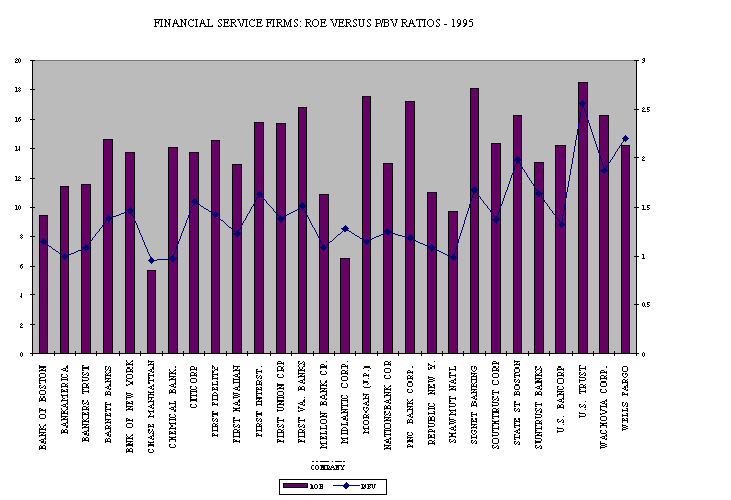A Simple Control for ROE ñ PBV / ROE

A simple way of controlling for differences in Return on Equity is to calculate the ratio of P/BV ratios to return on equity, and ranking firms on that basis. Using that approach, the financial service firms which had the lowest PBV/ROE ratios were ñ
 MOST OVERVALUED FIRMS MOST UNDERVALUED FIRMS Company Name ROE P/BV PBV/ROE Company Name ROE P/BV PBV/ROE MIDLANTIC CORP. 6.5 1.28 0.19692308 MORGAN (J.P.) 17.5 1.15 0.06571429 CHASE 5.7 0.95 0.16666667 PNC BANK CORP. 17.2 1.18 0.06860465 WELLS FARGO 14.2 2.2 0.15492958 CHEMICAL BANK. 14.1 0.97 0.06879433 U.S. TRUST 18.5 2.56 0.13837838 BANKAMERICA 11.4 1 0.0877193 SUNTRUST BANKS 13.1 1.64 0.12519084 FIRST UNION CRP 15.7 1.38 0.08789809

Estimating price-book value ratios from comparables

Year Regression R squared

1987 PBV = 0.1841 + .00200 PAYOUT - 0.3940 BETA + 1.3389 EGR + 9.35 ROE 0.8617

1988 PBV = 0.7113 + 0.00007 PAYOUT - 0.5082 BETA + 0.4605 EGR + 6.9374 ROE 0.8405

1989 PBV = 0.4119 + 0.0063 PAYOUT - 0.6406 BETA + 1.0038 EGR+ 9.55 ROE 0.8851

1990 PBV = 0.8124 + 0.0099 PAYOUT - 0.1857 BETA + 1.1130 EGR+ 6.61 ROE 0.8846

1991 PBV =1.1065 + 0.3505 PAYOUT - 0.6471 BETA + 1.0087 EGR + 10.51 ROE 0.8601

where,

PBV = Price / Book Value Ratio at the end of the year PAYOUT = Dividend Payout ratio at end of the year

BETA = Beta of the stock EGR = Growth rate in earnings over prior five years

ROE = Return on Equity = Net Income / Book Value of Equity

1995 Regression: PBV = 0.4138 + 0.3194 PAYOUT - 0.3418 BETA + 0.3549 EGR+ 14.75 ROE R2=0.7726

EGR = Expected earnings growth rate over next five years

Illustration 19 : Valuing aan initial public offering using the cross-sectional regression

Assume that you had been asked to value Semitool, a company which manufactures equipment used in the fabrication of semiconductors early in 1995 and that you had obtained the following data on the company:

Book Value of Equity = \$ 29.60 million

Net Income in 1994 = \$3.60 million

Beta based upon comparable firms = 1.40 (This is the average beta for comparable firms)

Free Cash Flow to Equity in 1994 = - 2.50 million

Expected growth rate over next five years = 20%

First compute the variables in the desired units,

Payout = 0% (Assume that this company will not pay dividends) Earnings Growth Rate = 20%

Return on Equity = 3.60/29.60 = 12.16% Beta = 1.40

Predicted PBV Ratio = 0.4138 + 0.3194(0) - 0.3418 (1.40) + 0.3549 (.20)+ 14.75 (.1216) = 1.80

Predicted Market Value of Equity in Semitool Inc. = 1.80 * \$ 29.60 million= \$53.28 million

Price-Book Value Ratios and Investment Strategies

Price-Book Value Ratios and Excess Returns

• Several studies have established a relationship between price-book value ratios and excess returns.
• The discussion in the preceding section emphasized the importance of return on equity in determining the price/book value ratio, and noted that only firms with high return on equity and low price-book value ratio could be considered undervalued.
• This proposition was tested by screening all NYSE stocks from 1981 to 1990, on the basis of both price-book value ratios and returns on equity and creating two portfolios - an 'undervalued' portfolio with low price-book value ratios (in bottom 25% of universe) and high returns on equity (in top 25% of universe) and an overvalued portfolio with high price-book value ratios (in top 25% of universe) and low returns on equity (in bottom 25%)- each year, and then estimating excess returns on each portfolio in the following year.
• The undervalued portfolio made an average return on 25.60% over the ten year period compared to the S&P 500 return of 17.49%. The overvalued portfolio made 10.61% annually.